# 些许吐槽

/ 0评 / 1

1、业务实现结构混乱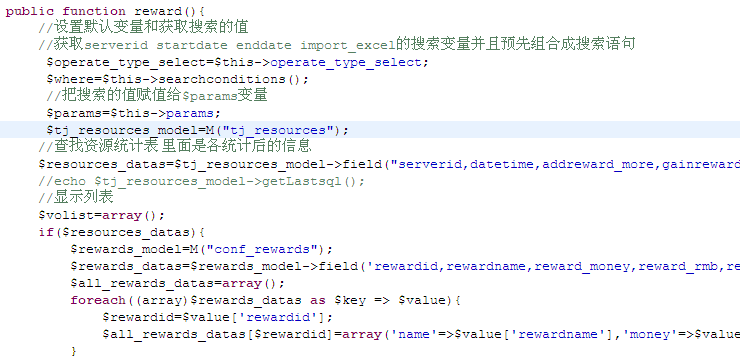ThinkPHP怎么说也是PHP5，ORM面向对象的开发框架。不过在这系统里面，Controller的业务处理作用几乎是100%，Model几乎被闲置，最最关键的是开发人员说“我觉得Model没有什么用”，我只能表示黑线了。而现在我尽可能的使用Model构建业务，Controller做转发和调用。

2、代码结构不分块，几乎没有组织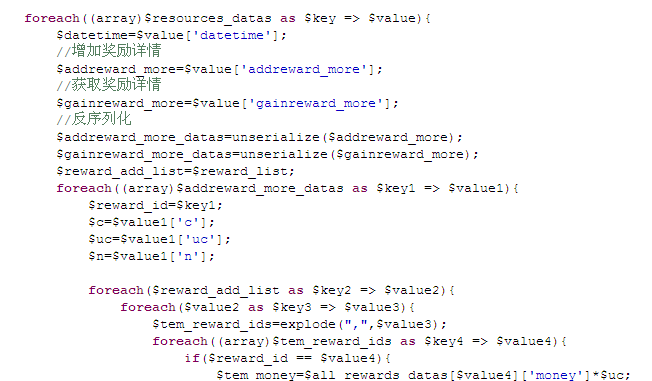3、代码非常冗余

```
//导出excel列表
\$import_excel=\$params['import_excel'];
if (\$import_excel == 1)
{
C ( 'SHOW_PAGE_TRACE', false );
}

```

```
/**
* 查询list导出为excel
* @param int \$import_excel
* @param string \$outname
* @author Lei
* @time 2013-10-10 13:56:30
*/
if (\$import_excel == 1) {
C ( 'SHOW_PAGE_TRACE', false );
}
}

```

4、条件分支被大量滥用

```\$table_name="";
if(\$this->partition=='1'){
\$table_name=C("DB_PREFIX").\$this->tablename.date("Ymd",strtotime(\$date));
if(empty(\$mksql_array[\$table_name])){
\$_makesql=str_replace("{TABLE_NAME}",\$table_name,\$this->_makesql);
\$sql = "select TABLE_NAME from INFORMATION_SCHEMA.TABLES where `TABLE_SCHEMA`='".C("DB_NAME")."' and  TABLE_NAME ='".\$table_name."'";
\$tablelist = \$model->query(\$sql);
if(empty(\$tablelist)){
\$model->query(\$_makesql);
}
\$mksql_array[\$table_name]=1;
}

}elseif(\$this->partition=='2'){
\$table_name=C("DB_PREFIX").\$this->tablename.date("Ym",strtotime(\$date));
if(empty(\$mksql_array[\$table_name])){

\$_makesql=str_replace("{TABLE_NAME}",\$table_name,\$this->_makesql);

\$sql = "select TABLE_NAME from INFORMATION_SCHEMA.TABLES where `TABLE_SCHEMA`='".C("DB_NAME")."' and  TABLE_NAME ='".\$table_name."'";
\$tablelist = \$model->query(\$sql);
if(empty(\$tablelist)){
\$model->query(\$_makesql);
}
\$mksql_array[\$table_name]=1;
}
}else{
\$table_name=C("DB_PREFIX").\$this->tablename;
if(empty(\$mksql_array[\$table_name])){
\$_makesql=str_replace("{TABLE_NAME}",\$table_name,\$this->_makesql);
\$sql = "select TABLE_NAME from INFORMATION_SCHEMA.TABLES where `TABLE_SCHEMA`='".C("DB_NAME")."' and  TABLE_NAME ='".\$table_name."'";
\$tablelist = \$model->query(\$sql);
if(empty(\$tablelist)){
\$model->query(\$_makesql);
}
\$mksql_array[\$table_name]=1;
}
\$table_name=C("DB_PREFIX").\$this->tablename;
}
```

```
/**
* 检测以及创建对应的logtable
*
* @param string \$date
* @return string
* @author Lei.Lu
*/
protected function createLogTable(\$date){
\$model = M ();
static \$mksql_array = array ();
\$table_name = "";

\$dbprefix = C ( "DB_PREFIX" );

switch (\$this->partition){
case '1':
\$table_name = \$dbprefix . \$this->tablename . date ( "Ymd", strtotime ( \$date ) );
break;
case '2':
\$table_name = \$dbprefix . \$this->tablename . date ( "Ym", strtotime ( \$date ) );
break;
default:
\$table_name = \$dbprefix . \$this->tablename;
}

if (empty ( \$mksql_array [\$table_name] )) {
\$_makesql = str_replace ( "{TABLE_NAME}", \$table_name, \$this->_makesql );
\$sql = "select TABLE_NAME from INFORMATION_SCHEMA.TABLES where `TABLE_SCHEMA`='" . C ( "DB_NAME" ) . "' and  TABLE_NAME ='" . \$table_name . "'";
\$tablelist = \$model->query ( \$sql );
if (empty ( \$tablelist )) {
\$model->query ( \$_makesql );
}
\$mksql_array [\$table_name] = 1;
}

return \$table_name;
}

```

5、各种不规范的地方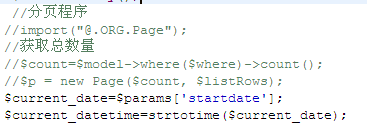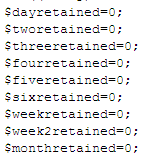╮(╯▽╰)╭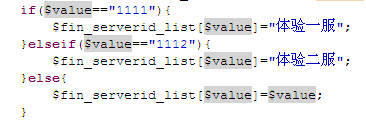• 默认
• 护眼
• 夜晚
• Serif
• Sans